• fft实验源码，希望对你有帮助，运行后有图，基于MATLAB的 数字图像处理 matlab源代码及解释
• 基于MATLAB的数字信号处理， 用FFT对信号作频谱分析。
文章目录一、实验目的二、实验原理与方法三、实验内容及步骤1. 有限长序列2. 周期序列3. 模拟周期信号四、回答思考题五、实验总结
一、实验目的
学习用 FFT 对连续信号和时域离散信号进行频谱分析（也称谱分析）的方法， 了解可能出现的分析误差及其原因，以便正确应用FFT。
二、实验原理与方法

用FFT对信号作频谱分析是学习数字信号处理的重要内容，经常需要进行谱分析的信号是模拟信号和时域离散信号，对信号进行谱分析的重要问题是频谱分辨率 D 和分析误差。
频谱分辨率直接和 FFT 的变换区间 N 有关，因为FFT能够实现的频率分辨率是2π/N，因此要求2π/N≤D。可以根据此式选择 FFT 的变换区间N。误差主要来自于用 FFT 作频谱分析时，得到的是离散谱，而信号（周期信号除外）是连续谱，只有当 N 较大时离散谱的包络才能逼近于连续谱，因此 N 要适当选择大一些。
周期信号的频谱是离散谱，只有用整数倍周期的长度作FFT，得到的离散谱才能代表周期信号的频谱。如果不知道信号周期，可以尽量选择信号的观察时间长一些。
对模拟信号进行谱分析时，首先要按照采样定理将其变成时域离散信号。如果是模拟周期信号，也应该选取整数倍周期的长度，经过采样后形成周期序列，按照周期序列的谱分析进行。

三、实验内容及步骤
1. 有限长序列选择 FFT 的变换区间 N 为 8 和 16 的两种情况进行频谱分析。分别打印其幅频特性曲线， 并进行对比、 分析和讨论。
%R4(n)的谱分析   有限长序列
%做N点DFT  不够的话  时域补零到N点
%8点->16点  相邻谱线间隔变密  离散谱的包络更接近于连续谱
clear;

x1=[1 1 1 1];
%8点DFT
N1=8;
xk=fft(x1,N1);   %计算x1(n)的8点DFT
subplot(221);
stem(0:N1-1,[x1 zeros(1,N1-4)],'.','g'); %时域补零到 8个点 绘图
xlabel('n');
ylabel('x(n)');
title('R4(n)');
axis([0 8 0 1.2]);

subplot(222);
stem(0:0.25:1.75,abs(xk),'.','g');
xlabel('\omega/\pi');
ylabel('幅度');
title('8点DFT的结果');
axis([0 2 0 4.5]);

%16点DTF
N2=16;
xk=fft(x1,N2);
subplot(223);
stem(0:N2-1,[x1 zeros(1,N2-4)],'.','r'); %时域补零到16个点 绘图
xlabel('n');
ylabel('x(n)');
title('R4(n)');
axis([0 16 0 1.2]);

subplot(224);
stem(0:0.125:1.875,abs(xk),'.','r');
xlabel('\omega/\pi');
ylabel('幅度');
title('16点DFT的结果');
axis([0 2 0 4.5]);

运行效果如下：%x2(n)的谱分析
%做N点DFT  不够的话  时域补零到N点

clear;

x2=[1 2 3 4 4 3 2 1];

%8点DFT
N1=8;
subplot(221);
stem(0:N1-1,x2,'.','g');
xlabel('n');
ylabel('x(n)');
title('x2(n)');
axis([0 8 0 4.5]);

xk=fft(x2,N1);
subplot(222);
stem(0:0.25:1.75,abs(xk),'.','g');   %归一化
xlabel('\omega/\pi');
ylabel('幅度');
title('8点DFT的结果');
axis([0 2 0 24]);

%16点DFT
N2=16;
subplot(223);
stem(0:N2-1,[x2 zeros(1,N2-8)],'.','r');  %时域补零到16点
xlabel('n');
ylabel('x(n)');
title('x2(n)');
axis([0 16 0 4.5]);

xk=fft(x2,N2);
subplot(224);
stem(0:0.125:1.875,abs(xk),'.','r');
xlabel('\omega/\pi');
ylabel('幅度');
title('16点DFT的结果');
axis([0 2 0 24]);


运行效果如下：%x3(n)的频谱分析
%做N点DFT  不够的话  时域补零到N点
clear;

x3=[4 3 2 1 1 2 3 4];

%8点DFT
N1=8;
subplot(221);
stem(0:N1-1,x3,'.','g');
xlabel('n');
ylabel('x(n)');
title('x3(n)');
axis([0 8 0 4.5]);
xk=fft(x3,N1);
subplot(222);
stem(0:0.25:1.75,abs(xk),'.','g');
xlabel('\omega/\pi');
ylabel('幅度');
title('8点DFT的结果');
axis([0 2 0 24]);

%16点DFT
N2=16;
subplot(223);
stem(0:N2-1,[x3 zeros(1,N2-8)],'.','r'); %时域补零到16点
xlabel('n');
ylabel('x(n)');
title('x3(n)');
axis([0 16 0 4.5]);
xk=fft(x3,N2);
subplot(224);
stem(0:0.125:1.875,abs(xk),'.','r');
xlabel('\omega/\pi');
ylabel('幅度');
title('16点DFT的结果');
axis([0 2 0 24]);

运行效果如下：观察可以发现：

由 MATLAB 绘图可以发现，N=8时，x2(n) 和 x3(n) 的幅频特性是相同的，因为x2(n)=x3((n-4))R8(n)，循环移位关系，所以 x3(n) 与 x2(n) 的 DFT 的幅频特性相同，如图 (2a) 和 (3a) 所示
但是，当 N=16时，x3(n) 与 x2(n) 就不满足循环移位关系了，所以如图 (2b) 和 (3b) 所示，幅频特性不同

2. 周期序列选择 FFT 的变换区间 N 为 8 和 16 的两种情况分别对以上序列进行频谱分析，分别打印其幅频特性曲线。 并进行对比、分析和讨论。
%x4(n)=cos(pi/4*n)的频谱分析   周期序列
%周期序列x(n)周期如果事先不知道 截取M点进行DFT  再将截取长度扩大一倍
%比较二者主谱差别 若满足分析误差要求  这两个都可以近似表示x(n)的频谱
%否则  继续将截取长度加倍  直到前后两次主谱差别满足误差要求
%幅度跟N有关  主瓣会变窄 旁瓣会增加  更接近于真实的频谱 幅度是冲激那样的 又窄又高
clear;

n=0:31;
x4=cos(pi/4*n);

%8点DFT
N1=8;
subplot(221);
stem(0:1:31,x4,'.','g');
xlabel('n');
ylabel('x(n)');
title('x4(n)');
axis([0 31 -1.2 1.2]);

xk=fft(x4,N1);
subplot(222);
stem(0:0.25:1.75,abs(xk),'.','g');
xlabel('\omega/\pi');
ylabel('幅度');
title('8点DFT的结果');
axis([0 2 0 5]);

%16点DFT
N2=16;
subplot(223);
stem(0:1:31,x4,'.','r');
xlabel('n');
ylabel('x(n)');
title('x4(n)');
axis([0 31 -1.2 1.2]);

xk=fft(x4,N2);
subplot(224);
stem(0:0.125:1.875,abs(xk),'.','r');
xlabel('\omega/\pi');
ylabel('幅度');
title('16点DFT的结果');
axis([0 2 0 9]);

运行效果如下：%x5(n)=cos(pi/4*n)+cos(pi/8*n)的频谱分析
%周期序列x(n)周期如果事先不知道 截取M点进行DFT  再将截取长度扩大一倍
%比较二者主谱差别满足分析误差要求  这两个都可以近似表示x(n)的频谱
%否则  继续将截取长度加倍  直到前后两次主谱差别满足误差要求
clear;

n=0:31;
x5=cos(pi/4*n)+cos(pi/8*n);

%8点DFT
N1=8;
subplot(321);
stem(0:1:31,x5,'.','m');
xlabel('n');
ylabel('x(n)');
title('x5(n)');
axis([0 31 -2.2 2.5]);

xk=fft(x5,N1);
subplot(322);
stem(0:0.25:1.75,abs(xk),'.','m');
xlabel('\omega/\pi');
ylabel('幅度');
title('8点DFT的结果');
axis([0 2 0 7]);

%16点DFT
N2=16;
subplot(323);
stem(0:1:31,x5,'.','r');
xlabel('n');
ylabel('x(n)');
title('x5(n)');
axis([0 31 -2.2 2.5]);

xk=fft(x5,N2);
subplot(324);
stem(0:0.125:1.875,abs(xk),'.','r');
xlabel('\omega/\pi');
ylabel('幅度');
title('16点DFT的结果');
axis([0 2 0 10]);

%32点DFT
N2=32;
subplot(325);
stem(0:1:31,x5,'.','g');
xlabel('n');
ylabel('x(n)');
title('x5(n)');
axis([0 31 -2.2 2.5]);

xk=fft(x5,N2);
subplot(326);
stem(0:0.0625:1.9375,abs(xk),'.','g');
xlabel('\omega/\pi');
ylabel('幅度');
title('32点DFT的结果');
axis([0 2 0 20]);

运行效果如下：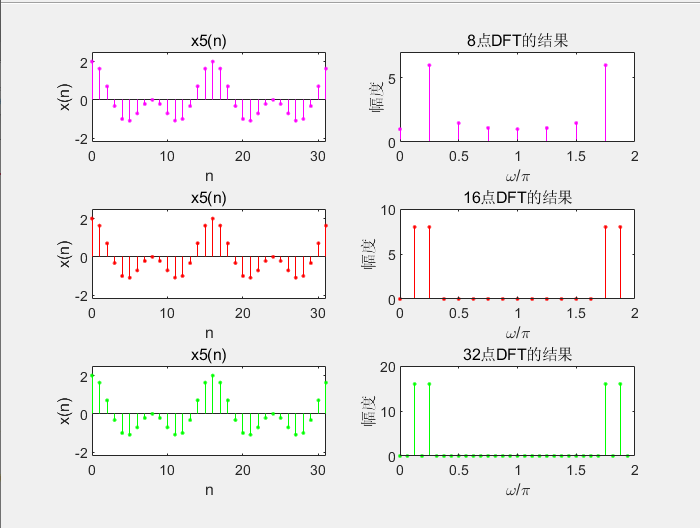对周期序列 x(n) 进行谱分析，如果事先不知道周期，可以先截取 M 点进行DFT ，再将截取长度扩大一倍，比较结果，如果二者的差别满足分析误差要求，则可以近似表示该信号的频谱，如果不满足误差要求就继续将截取长度加倍，重复比较，直到结果满足要求。
幅度为N/2，N增大，主瓣会变窄，旁瓣会增加 ，更接近于真实的频谱，幅度是冲激那样的，又窄又高。

3. 模拟周期信号%对模拟周期信号作谱分析
%首先要按照采样定理将其变成时域离散信号
%如果是模拟周期信号， 也应该选取整数倍周期的长度， 经过采样后形成周期序列
%再按照周期序列的谱分析进行
clear;

Fs=64;T=1/Fs;

%先按照采样定理将模拟信号变成时域离散信号
N=16;n=0:N-1; %FFT的变换区间 N=16
x6nT=cos(8*pi*n*T)+cos(16*pi*n*T)+cos(20*pi*n*T); %对x6(t) 16点采样

%fftshift移动零频点到频谱中间 为了把结果和fft运算的结果一致
X6k16=fftshift(fft(x6nT,16)); %计算 x6nT 的16点 DFT
Tp=N*T;F=1/Tp;    %频率分辨率 F
k=0:N-1;fk1=-32:4:28; %产生16点 DFT 对应的采样点频率（以零频率为中心）
subplot(3,1,1);stem(fk1,abs(X6k16),'.','g'); %绘制16点DFT的幅频特性图
title('16点 DFT[x_6(nT)]|');xlabel('\omega/\pi');ylabel('幅度');
axis([-32 28 0 12]);

N=32;n=0:N-1; %FFT 的变换区间 N=32
x6nT=cos(8*pi*n*T)+cos(16*pi*n*T)+cos(20*pi*n*T); %对 x6(t) 32点采样
X6k32=fftshift(fft(x6nT,32)); %计算x6(nT)的 32 点 DFT
Tp=N*T;F=1/Tp;    %频率分辨率 F
k=0:N-1;fk2=-32:2:30; %产生 32 点 DFT 对应的采样点频率（以零频率为中心）
subplot(3,1,2);stem(fk2,abs(X6k32),'.','r'); %绘制 32 点 DFT 的幅频特性图
title('32点 DFT[x_6(nT)]|');xlabel('\omega/\pi');ylabel('幅度');
axis([-32 31 0 20]);

N=64;n=0:N-1; %FFT 的变换区间 N=64
x6nT=cos(8*pi*n*T)+cos(16*pi*n*T)+cos(20*pi*n*T); %对x6(t) 64点采样
X6k64=fftshift(fft(x6nT,64)); %计算 x6(nT) 的64点DFT
Tp=N*T;F=1/Tp;    %频率分辨率 F
k=0:N-1;fk3=-32:1:31; %产生64点 DFT对应的采样点频率（以零频率为中心）
subplot(3,1,3);stem(fk3,abs(X6k64),'.','m'); %绘制64点DFT的幅频特性图
title('64点 DFT[x_6(nT)]|');xlabel('\omega/\pi');ylabel('幅度');
axis([-32 31 0 40]);


运行效果如下：四、回答思考题
（1） 对于周期序列， 如果周期不知道， 如何用 FFT 进行谱分析？
答：周期信号的周期预先不知道时，可先截取 M 点进行DFT，再将截取长度扩大一倍截取，比较结果，如果二者的差别满足分析误差要求,则可以近似表示该信号的频谱，如果不满足误差要求就继续将截取长度加倍，重复比较，直到结果满足要求。
（2） 如何选择FFT的变换区间（包括非周期信号和周期信号）？
答：对于非周期信号：有频谱分辨率F，而频谱分辨率直接和 FFT 的变换区间有关，因为 FFT 能够实现的频率分辨率是2π/N…因此有最小的N>2π/F。就可以根据此式选择 FFT 的变换区间。对于周期信号，周期信号的频谱是离散谱，只有用整数倍周期的长度作FFT，得到的离散谱才能代表周期信号的频谱。
（3）当 N=8 时， x2 (n) 和 x3 (n)的幅频特性会相同吗？为什么？N=16时呢？

由 MATLAB 绘图可以发现，N=8时，x2(n) 和 x3(n) 的幅频特性是相同的，因为x3(n)=x2((n+4))R8(n)，为循环移位关系，所以 x3(n) 与 x2(n) 的DFT的幅频特性相同，如图 (2a) 和 (3a) 所示
但是，当 N=16 时，x3(n) 与 x2(n) 就不满足循环移位关系了，所以如图 (2b) 和 (3b) 所示，幅频特性不同

五、实验总结

用 FFT 对信号作频谱分析是学习数字信号处理的重要内容，经常需要进行谱分析的信号是模拟信号和时域离散信号，对信号进行谱分析的重要问题是频谱分辨率 D 和分析误差。
频谱分辨率直接和 FFT 的变换区间 N 有关，因为FFT能够实现的频率分辨率是2π/N，因此要求2π/N≤D。可以根据此式选择 FFT 的变换区间N。误差主要来自于用 FFT 作频谱分析时，得到的是离散谱，而信号（周期信号除外）是连续谱，只有当 N 较大时离散谱的包络才能逼近于连续谱，因此 N 要适当选择大一些。
周期信号的频谱是离散谱，只有用整数倍周期的长度作FFT，得到的离散谱才能代表周期信号的频谱。如果不知道信号周期，可以尽量选择信号的观察时间长一些。
对模拟信号进行谱分析时，首先要按照采样定理将其变成时域离散信号。如果是模拟周期信号，也应该选取整数倍周期的长度，经过采样后形成周期序列，按照周期序列的谱分析进行。


展开全文MATLAB
• 基于C++实现FFT ——数字信号处理 ，现成的序列有：正弦 三角 矩形 采样
• 数字信号处理实验，包含代码跟实验截图，注释清晰明了，实验结果正确matlab
• (1) 进一步加深DFT算法原理和基本性质的理解(因为FFT只是DFT的一种快速算法，所以FFT的运算结果必然满足... (3) 学习利用FFT对离散时间信号进行频谱分析的方法，了解可能出现的误差及其原因，以便在实际中正确应用FFT
数字信号处理（一）利用FFT对信号进行频谱分析
1．实验目的
(1) 进一步加深DFT算法原理和基本性质的理解(因为FFT只是DFT的一种快速算法，所以FFT的运算结果必然满足DFT的基本性质)。
(2) 熟悉FFT算法原理和FFT程序的应用。
(3) 学习利用FFT对离散时间信号进行频谱分析的方法，了解可能出现的误差及其原因，以便在实际中正确应用FFT。
2．实验原理
本实验的原理DFT算法及其相关的基本性质，同时学习DFT的快速算法FFT。对于在数字信号处理之中占有重要地位的有限长序列来说，可以利用傅里叶变换和Z变换来进行处理。除此之外，针对有限长这个特点，可以导出一种更有利的工具—离散傅里叶变换
离散傅里叶变换从理论上解决了傅里叶变换应用于实际的可能性，但若直接按DFT公式来计算，运算量实在太大（与N的平方成比例）。快速傅里叶变换是离散傅里叶变换的快速算法，它大大减少了离散傅里叶变换的运算量，一般可以缩短一二个数量级，且N越大改善效果越明显，从而真正的让DFT的运算在实际工作中真正广泛应用。
对于所给出的有限长序列，逐个进行相应点数的离散傅立叶变换，可以得到该序列的频谱图，并进行相应的理论分析。
3．实验内容
（1）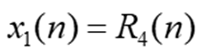分别做8点DFT和16点DFT，结果如下
图一 x1的8点DFT结果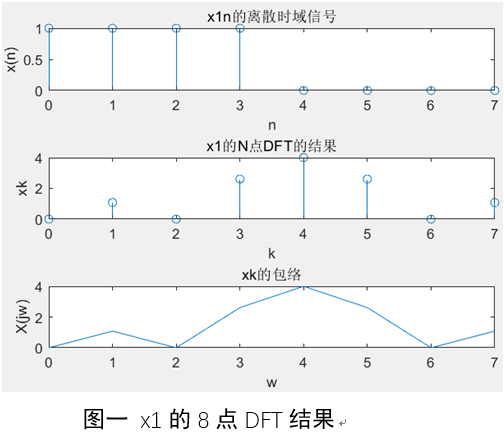如图为X1n的8点DFT频谱分析，图中从上到下分别显示的是x1n的离散时域信号，8点DFT结果以及8点DFT的包络，补了4个0之后做的8点DFT。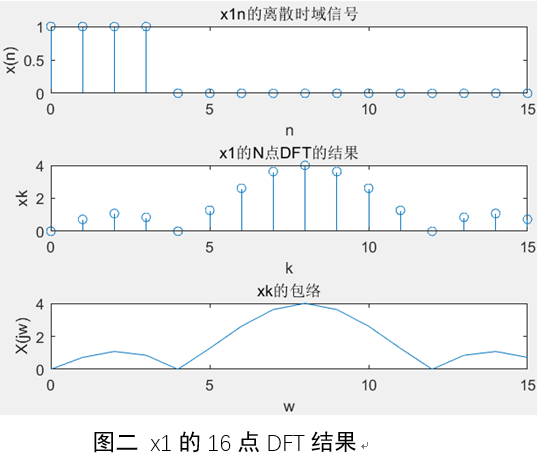图二 x1的16点DFT结果
如图所示是对X1n的16点DFT，由于X1n只有4个数据点，相当于在离散时域信号后面补了12个0之后做的16点DFT。
通过实验所得图像分析可得， 为矩形窗函数，其离散傅立叶变换为sa函数的包络，且图像关于中心对称分布。
（2）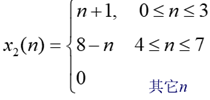分别做8点和16点DFT，结果如下
图三 x2的8点DFT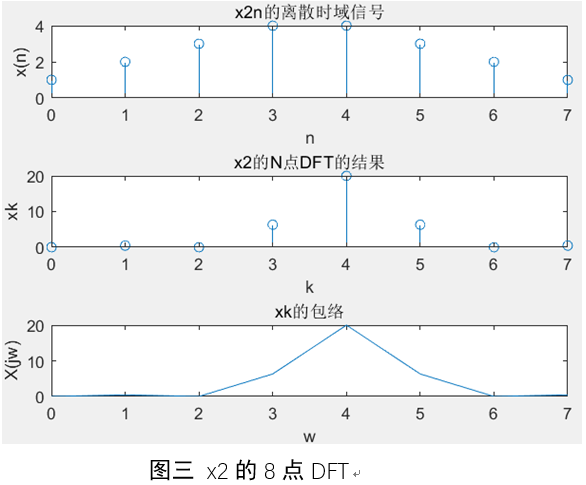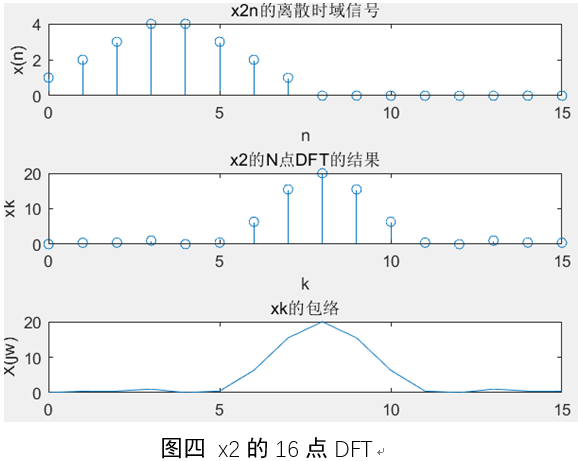图四 x2的16点DFT
通过实验所得图像分析可得， 为三角波，其离散傅立叶变换为sa函数平方的包络，且图像关于中心对称分布。
（3）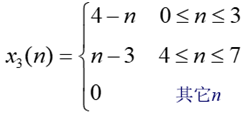的8点DFT和16点DFT对于所给函数进行离散傅立叶变化，画出信号的时域和频域波形如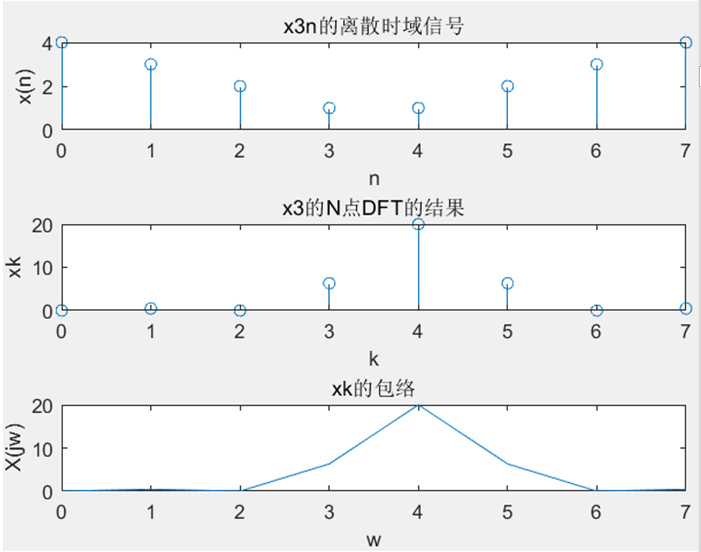图五 x3的8点DFT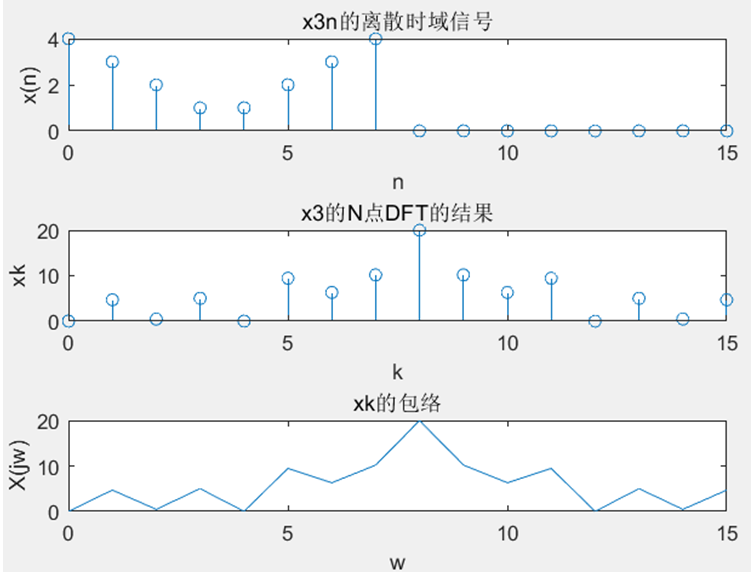图六 x3的16点DFT
（4）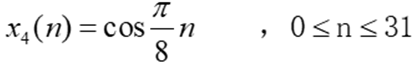的16点DFT和32点DFT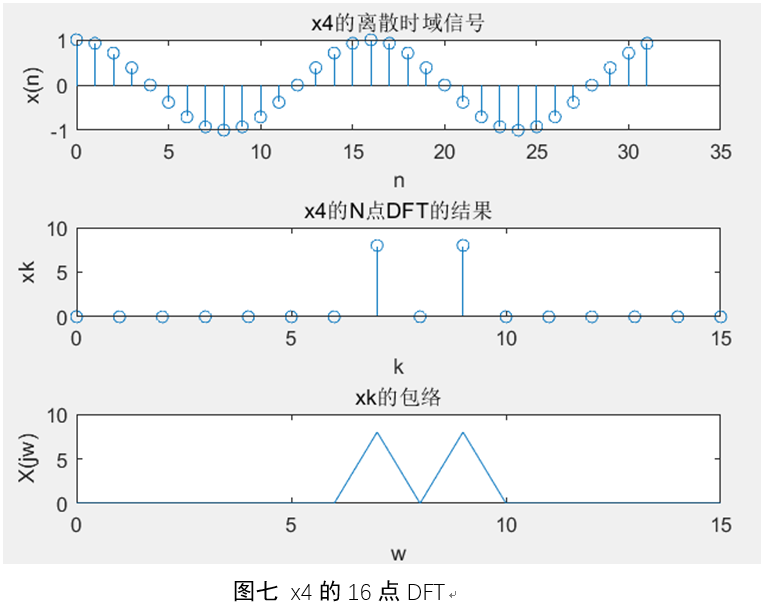图七 x4的16点DFT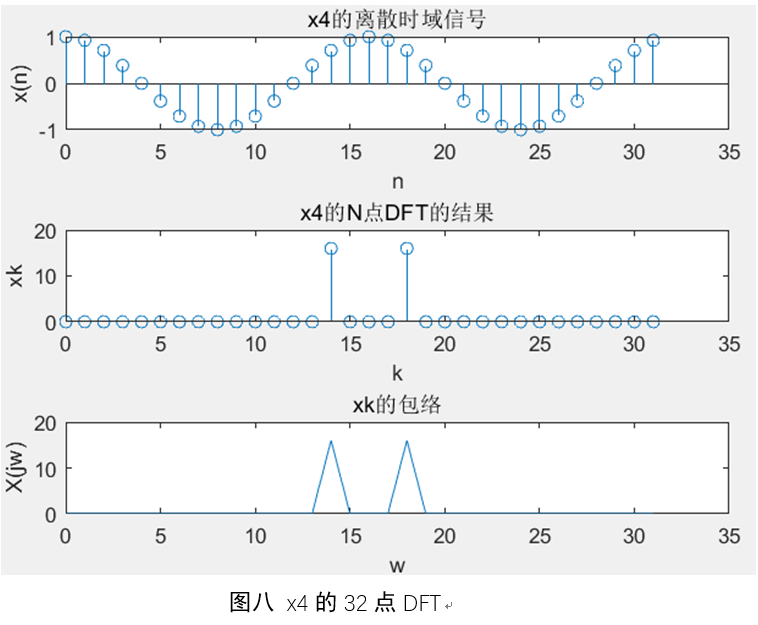图八 x4的32点DFT
通过实验所得图像分析可得， 为余弦波，其离散傅立叶变换为频率为关于 对称的两个冲激。
（5）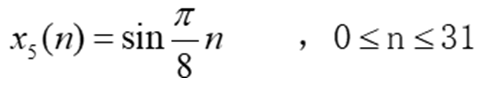的16和32点DFT，结果如下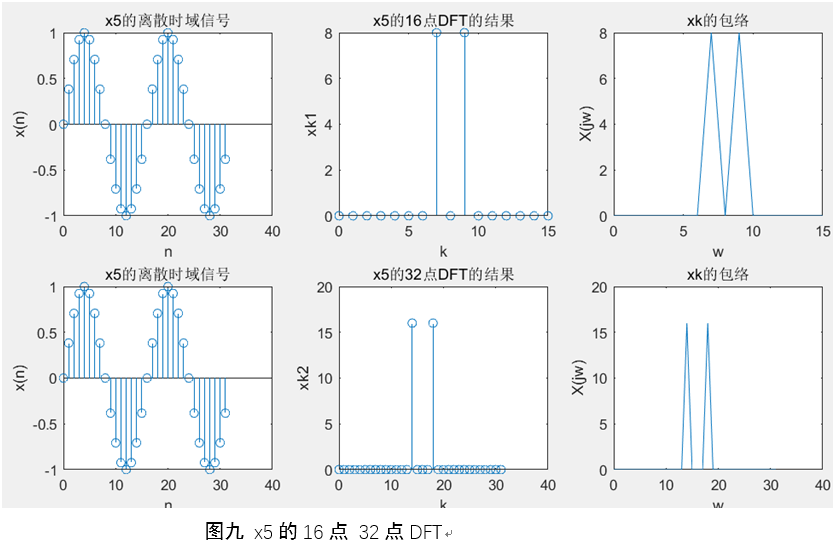图九 x5的16点 32点DFT
（6）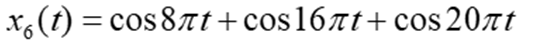处理方式一：采样率fs=64Hz,  分别做N=16, 32, 64点DFT  ，结果如下：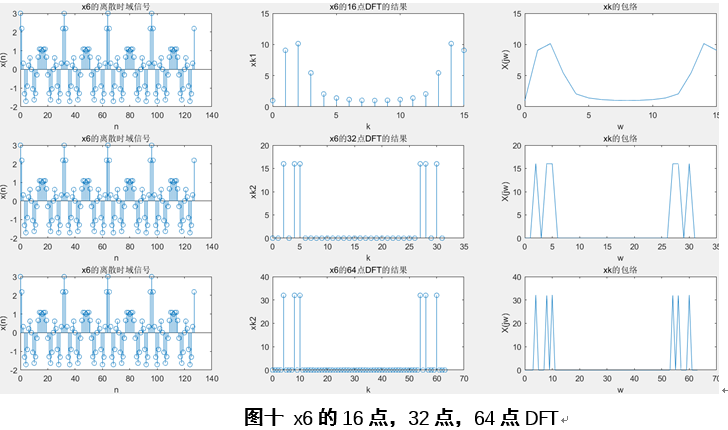观察我们的信号，不难看出应该是有三个频率分量，4Hz,8Hz,10Hz
由于我们选择相同的采样率，都取128个数据点，因此三种情况下我们抽样所得到的离散时域信号是相同的（反映在图中是左侧三个图像一致的），
A. 当做16点DFT时，由于选择的采样率是64Hz,则频域频谱间隔为4Hz,可以观察到图中二图的第二，三根谱线，对应的就是4Hz和8Hz,而10Hz的频率并不在我们的频率刻度线上，因此实际上是观测不到的，但我们确实看到第4，5，6根谱线确实不为0，是因为他们反映了图形的包络，其实就是上课的时候讲到的假包络
B. 当我们做32点DFT时，由于选择的采样率是64Hz,则对应的频谱间隔为2Hz,这样我们可以在DFT结果之中真实准确的看到在前半个周期内第2，4，5根谱线是有值的，后半个周期是对称的；
C. 当我们做64点DFT时，由于选择的采样率是64Hz,则对应的频谱间隔为1Hz,这样我们可以在DFT结果之中真实准确的看到在前半个周期内第4，8，10根谱线是有值的，后半个周期是对称的；
（7）	令x(n)=x4(n)+jx5(n)，
对x(n)计算16点、32点和 64点 (时域补零)的快速傅里叶变换，即X(k)=FFT［x(n)］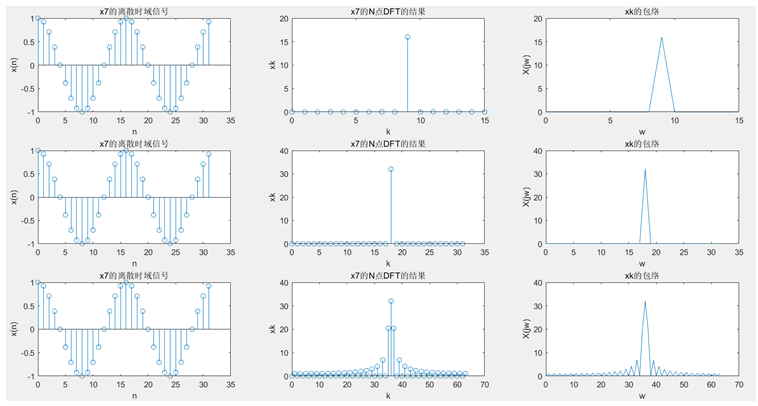通过实验所得图像分析可得， 为离散复信号，用欧拉公式可化简为 。而可知复信号只有正频率，没有负频率，因此对其进行离散傅立叶变化后得到中心为 的sa函数冲激。且对函数的补零越多，其sa函数包络越明显。
4．思考题
问题一： 在N=8时， x2(n)和x3(n)的幅频特性会相同吗? 为什么? N=16时呢?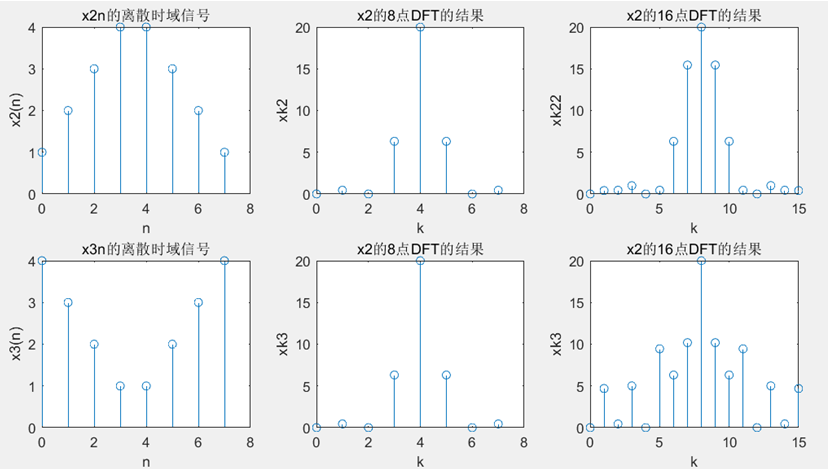当N=8时， 的 幅频特性不完全相同，它们的幅度特性相同，但是相位相差 相角。因为 进行8点周期延拓后的周期函数，平移4点后截短就是 。而时域的平移对应频域的相位线性变化，所以它们会有 的相位差。
当N=16是， 的 进行了时域补零，所对应的周期函数没有什么关系。所以它们的幅频特性不同。
问题二：(2) 对一个周期序列分别取一个周期或多个周期所作的频谱分析结果是否有区别?
有区别，因为对于一个周期和多个周期的频谱分析，其取样时矩形函数的宽度不同，则频域所对应的包络的宽度也不同。当所取的周期越长，其取样时矩形函数的宽度越宽，频域卷积的sa函数的宽度也越窄，越接近理想频谱。
5．个人感想与总结：

通过“利用FFT对信号进行频谱分析”的实验，我掌握了利用Matlab对信号做FFT进行频域分析的方法。通过实验中，不同的函数离散傅立叶变换的结果，并对结果进行理论分析，我对于离散傅立叶变换的性质有了更加深入的理解。对于离散傅立叶变化与连续时间傅立叶变换的关系，也更加清晰了。
同时，在实验过程之中，在编程实际中，通过学习书后的代码，和网上共享的资料，我渐渐地对MATLAB编程熟悉起来，而且编程思维和编程能力都有大幅提高，以后在漫长的学习生活之中，相信对matlab的实用会更加驾轻就熟，使得工程实际的效率进一步的提高。
在实验后，对所得到的实验结果进一步分析，尤其是对不同采样率，不同DFT点数，不同数据点数下DFT的结果的认识更加清楚，对上课的时候老师讲的三个同学也有更深的体会。另外对于周期函数的抽样时间长度对于频域的卷积sa函数的宽度的影响，抽样后的周期函数与理想的周期函数的傅立叶变换之间的联系与不同等等。
这次实验让我独立认识问题，思考问题，解决问题，攻关克难的能力都有大的提升。

6. 附录：程序代码：
x1=[1 1 1 1];
N=8;
xk=fftshift(fft(x1,N));
subplot(3,1,1);%用于分割
stem(0:N-1,[x1 zeros(1,N-4)]);
xlabel('n');
ylabel('x(n)');
title('x1n的离散时域信号');
subplot(3,1,2);
stem(0:N-1,abs(xk));
xlabel('k');
ylabel('xk');
title('x1的N点DFT的结果');
subplot(3,1,3);
plot(0:N-1,abs(xk));
xlabel('w');
ylabel('X(jw)');
title('xk的包络');

x2=[1 2 3 4 4 3 2 1];
N=8;
xk=fftshift(fft(x2,N));
subplot(3,1,1);%用于分割
stem(0:N-1,[x2 zeros(1,N-8)]);
xlabel('n');
ylabel('x(n)');
subplot(3,1,2);
title('x2n的离散时域信号');
stem(0:N-1,abs(xk));
xlabel('k');
ylabel('xk');
title('x2的N点DFT的结果');
subplot(3,1,3);
plot(0:N-1,abs(xk));
xlabel('w');
ylabel('X(jw)');
title('xk的包络');

x3=[4 3 2 1 1 2 3 4];
N=8;
xk=fftshift(fft(x3,N));
subplot(3,1,1);%用于分割
stem(0:N-1,[x3,zeros(1,N-8)]);
xlabel('n');
ylabel('x(n)');
title('x3n的离散时域信号');
subplot(3,1,2);
stem(0:N-1,abs(xk));
xlabel('k');
ylabel('xk');
title('x3的N点DFT的结果');
subplot(3,1,3);
plot(0:N-1,abs(xk));
xlabel('w');
ylabel('X(jw)');
title('xk的包络');

n=[0:31];
x4=cos(pi/8*n);
N=16;
xk=fftshift(fft(x4,N));
subplot(3,1,1);%用于分割
stem(0:31,x4);
xlabel('n');
ylabel('x(n)');
title('x4的离散时域信号');
subplot(3,1,2);
stem(0:N-1,abs(xk));
xlabel('k');
ylabel('xk');
title('x4的N点DFT的结果');
subplot(3,1,3);
plot(0:N-1,abs(xk));
xlabel('w');
ylabel('X(jw)');
title('xk的包络');

n=[0:31];
x4=cos(pi/8*n);
N=32;
xk=fftshift(fft(x4,N));
subplot(3,1,1);%用于分割
stem(0:31,x4);
xlabel('n');
ylabel('x(n)');
title('x4的离散时域信号');
subplot(3,1,2);
stem(0:N-1,abs(xk));
xlabel('k');
ylabel('xk');
title('x4的N点DFT的结果');
subplot(3,1,3);
plot(0:N-1,abs(xk));
xlabel('w');
ylabel('X(jw)');
title('xk的包络');

n=[0:31];
x5=sin(pi/8*n);
xk1=fftshift(fft(x5,16));
xk2=fftshift(fft(x5,32));
subplot(2,3,1);
stem(0:31,x5);
xlabel('n');
ylabel('x(n)');
title('x5的离散时域信号');
subplot(2,3,2);
stem(0:15,abs(xk1));
xlabel('k');
ylabel('xk1');
title('x5的16点DFT的结果');
subplot(2,3,3);
plot(0:15,abs(xk1));
xlabel('w');
ylabel('X(jw)');
title('xk的包络');

subplot(2,3,4);
stem(0:31,x5);
xlabel('n');
ylabel('x(n)');
title('x5的离散时域信号');
subplot(2,3,5);
stem(0:31,abs(xk2));
xlabel('k');
ylabel('xk2');
title('x5的32点DFT的结果');
subplot(2,3,6);
plot(0:N-1,abs(xk2));
xlabel('w');

fs=64;
delta_t=1/fs;
N=128;
t=[0:N-1]*delta_t;
x6=cos(8*pi*t)+cos(16*pi*t)+cos(20*pi*t);
xk1=fft(x6,16);
xk2=fft(x6,32);
xk3=fft(x6,64);
subplot(3,3,1);
stem(0:127,x6);
xlabel('n');
ylabel('x(n)');
title('x6的离散时域信号');
subplot(3,3,2);
stem(0:15,abs(xk1));
xlabel('k');
ylabel('xk1');
title('x6的16点DFT的结果');
subplot(3,3,3);
plot(0:15,abs(xk1));
xlabel('w');
ylabel('X(jw)');
title('xk的包络');

subplot(3,3,4);
stem(0:127,x6);
xlabel('n');
ylabel('x(n)');
title('x6的离散时域信号');
subplot(3,3,5);
stem(0:31,abs(xk2));
xlabel('k');
ylabel('xk2');
title('x6的32点DFT的结果');
subplot(3,3,6);
plot(0:31,abs(xk2));
xlabel('w');
ylabel('X(jw)');
title('xk的包络');

subplot(3,3,7);
stem(0:127,x6);
xlabel('n');
ylabel('x(n)');
title('x6的离散时域信号');
subplot(3,3,8);
stem(0:63,abs(xk3));
xlabel('k');
ylabel('xk2');
title('x6的64点DFT的结果');
subplot(3,3,9);
plot(0:63,abs(xk3));
xlabel('w');
ylabel('X(jw)');

n=[0:31];
x7=cos(pi/8*n)+j*sin(pi/8*n);
xk1=fftshift(fft(x7,16));
xk2=fftshift(fft(x7,32));
xk3=fftshift(fft(x7,64));
subplot(3,3,1);%用于分割
stem(0:31,x7);
xlabel('n');
ylabel('x(n)');
title('x7的离散时域信号');
subplot(3,3,2);
stem(0:15,abs(xk1));
xlabel('k');
ylabel('xk');
title('x7的16点DFT的结果');
subplot(3,3,3);
plot(0:15,abs(xk1));
xlabel('w');
ylabel('X(jw)');
title('xk的包络');

subplot(3,3,4);%用于分割
stem(0:31,x7);
xlabel('n');
ylabel('x(n)');
title('x7的离散时域信号');
subplot(3,3,5);
stem(0:31,abs(xk2));
xlabel('k');
ylabel('xk');
title('x7的32点DFT的结果');
subplot(3,3,6);
plot(0:31,abs(xk2));
xlabel('w');
ylabel('X(jw)');
title('xk的包络');

subplot(3,3,7);%用于分割
stem(0:31,x7);
xlabel('n');
ylabel('x(n)');
title('x7的离散时域信号');
subplot(3,3,8);
stem(0:63,abs(xk3));
xlabel('k');
ylabel('xk');
title('x7的64点DFT的结果');
subplot(3,3,9);
plot(0:63,abs(xk3));
xlabel('w');

x2=[1 2 3 4 4 3 2 1];
x3=[4 3 2 1 1 2 3 4];
N1=8;
N2=16;
xk21=fftshift(fft(x2,N1));
xk31=fftshift(fft(x3,N1));
xk22=fftshift(fft(x2,N2));
xk32=fftshift(fft(x3,N2));

subplot(2,3,1);%用于分割
stem(0:N1-1,[x2,zeros(1,N1-8)]);
xlabel('n');
ylabel('x2(n)');
title('x2n的离散时域信号');

subplot(2,3,2);
stem(0:N1-1,abs(xk21));
xlabel('k');
ylabel('xk2');
title('x2的8点DFT的结果');

subplot(2,3,3);
stem(0:N2-1,abs(xk22));
xlabel('k');
ylabel('xk22');
title('x2的16点DFT的结果');

subplot(2,3,4);
stem(0:N1-1,[x3,zeros(1,N1-8)]);
xlabel('n');
ylabel('x3(n)');
title('x3n的离散时域信号');

subplot(2,3,5);
stem(0:N1-1,abs(xk31));
xlabel('k');
ylabel('xk3');
title('x2的8点DFT的结果');

subplot(2,3,6);
stem(0:N2-1,abs(xk32));
xlabel('k');
ylabel('xk3');



展开全文快速傅里叶变换 信号频谱分析 matlab系统仿真
• C++实现任意点数的FFT及IFFT（数字信号处理 FFT）。 包含复数类和FFT实现类，mainfft.cpp为实例程序。 数字信号处理 FFT
• 数字信号处理课本 超星视频：B站视频链接 第四章 FFT 4.1 DFT运算量和FFT运算量对比 DFT：N2N^2N2次复乘，N(N−1)N(N-1)N(N−1)次复加 FFT：N2log2N\frac{N}{2}log_2N2N​log2​N次复乘，Nlog2NNlog_2NNlog2​N次...
作者：BerenCamlost
参考资料：

老师PPT：百度网盘链接 提取码: dhn4
数字信号处理课本
超星视频：B站视频链接

第四章 FFT
4.1 DFT运算量和FFT运算量对比

DFT：$N^2$次复乘，$N(N-1)$次复加
FFT：$\frac{N}{2}log_2N$次复乘，$Nlog_2N$次复加

其中FFT中的N为2的整次幂

4.2 按时间抽取的基2 - FFT原理
4.2.1 算法原理
$\left\{\begin{matrix} X[k]=G[k]+W_N^kH[k] & k=0,1,2...\frac{N}{2}-1\\ X[k+\frac{N}{2}]=G[k]-W_N^kH[k] & k=0,1,2...\frac{N}{2}-1 \end{matrix}\right.$

其中N为2的整次幂

4.2.2 蝶形算法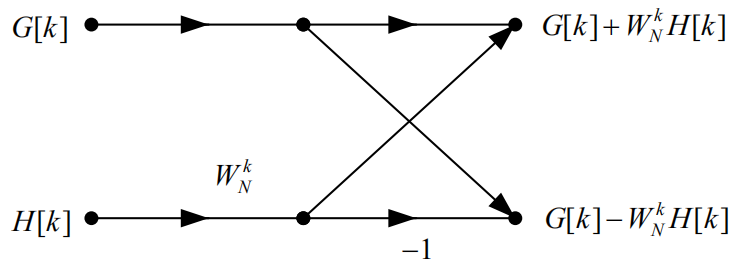蝶形算法和上面的公式是同一个东西的不同表现形式。

4.3 按时间抽取的FFT流图

这里以16点FFT流图为例进行解释（注意这个图的WN标注的位置可能有些奇怪）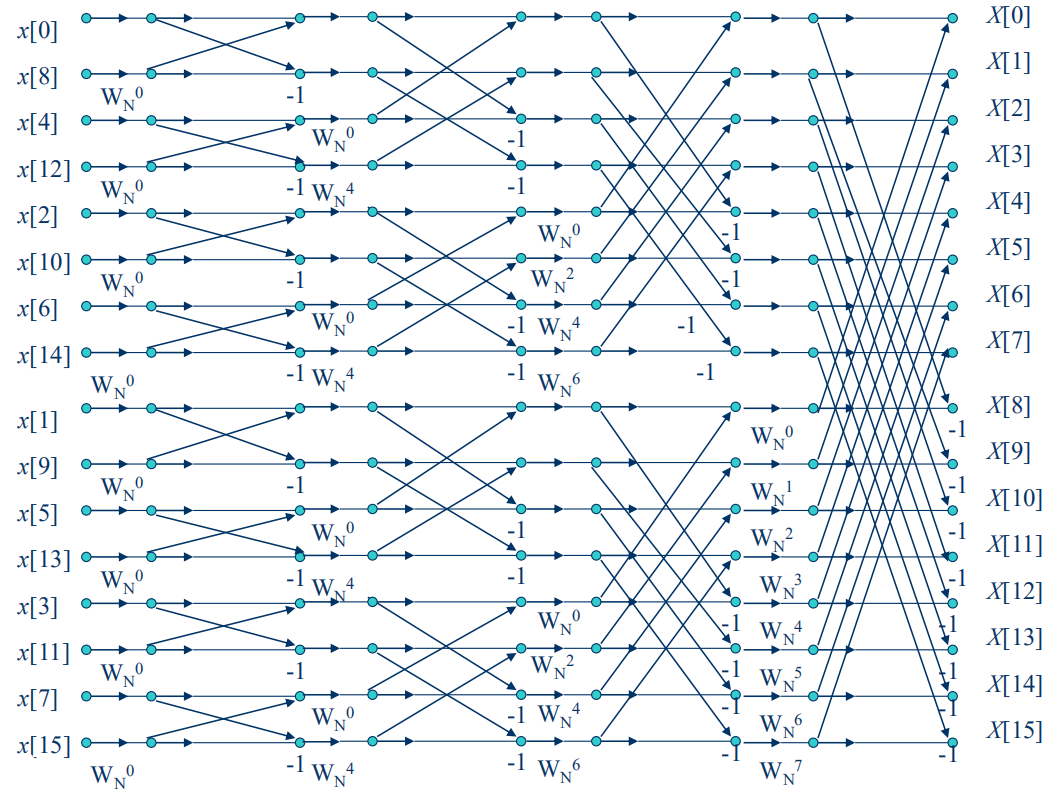4.3.1 算法原理
由蝶形构成基本运算单元，每个流图共有M级，每一竖列有N/2个蝶形，于是得到公式$M=log_2N$
4.3.2 原位运算
由于DFT算法的限制，导致源数据将作为每一次运算的操作数，这使得储存容量的要求很大。而FFT算法，从蝶形图中可以看出，下一个蝶形的数据源和上一次的数据源是不同的，所以可以把每一次蝶形运算得到的数据直接存放在原来的存储区域，大大减小了存储容量的要求。
4.3.3 序数重排
可以看到，FFT的结果值是按顺序排列的，如果把计算结果k的二进制和数据源的n的二进制相比较，可以发现如下规律：

数据源顺序
二进制
二进制
FFT顺序

0
0000
0000
0

8
1000
0001
1

4
0100
0010
2

12
1100
0011
3

2
0010
0100
4

10
1010
0101
5

6
0110
0110
6

14
1110
0111
7

等等……
可以发现前后两个数的二进制是正好反过来的。
4.3.4 系数的确定
随缘


展开全文• ## FFT_频谱分析（数字信号处理）

万次阅读 多人点赞 2018-12-10 16:45:15
FFT_频谱分析（数字信号处理） （一）实验原理 用FFT对信号作频谱分析是学习数字信号处理的重要内容。经常需要进行谱分析的信号是模拟信号和时域离散信号。对信号进行谱分析的重点在于频谱分辨率及分析误差。频谱...
~~ 如果有什么问题可以在我的个人博客留言 ，我会及时回复。欢迎来访交流 ~~

FFT_频谱分析（数字信号处理）

（一）实验原理

用FFT对信号作频谱分析是学习数字信号处理的重要内容。经常需要进行谱分析的信号是模拟信号和时域离散信号。对信号进行谱分析的重点在于频谱分辨率及分析误差。频谱分辨率D和频谱分析的点数N直接相关，其分辨率为2π/N 。因此2π/N≤D，可以据这个公式确定频率的分辨率。

FFT分析频谱的误差在于得到的是离散谱，而信号（非周期信号）是连续谱，只有当N较大时，离散谱的包络才能逼近于连续谱。因此N要适当选择大一些。

周期信号的频谱是离散谱，只有用整数倍周期的长度作FFT，得到的离散谱才能代表周期信号的频谱。如果不知道信号周期，可以尽量选择信号的观察时间长一些。

对模拟信号进行谱分析时，首先要按照采样定理将其变成时域离散信号。如果是模拟周期信号，也应该选取整数倍周期的长度，经过采样后形成周期序列，按照周期序列的谱分析进行。

（二）实验步骤

1.对以下序列进行频谱分析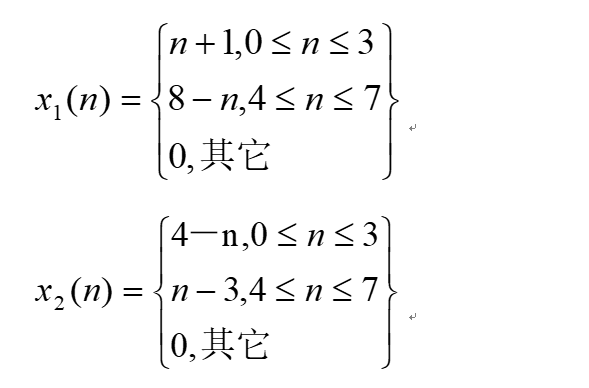为上三角有限长序列，序列长度为8； 为下三角有限长序列，序列长度为8。选择FFT变换点数N分别为8和16两种情况进行频谱分析，打印出频谱特性曲线，观察不同N值， 和 的频谱特性曲线是否相同，进行讨论分析并得出结论。整个频谱分析过程通过Matlab软件进行程序设计实现。以下为Matlab的程序实现：

function e_6_1
M=8;xa=1:(M/2);xb=(M/2):-1:1;x1n=[xa,xb]; x2n=[xb,xa];%产生长度为M的上下三角序列
subplot(321);stem([0:M-1],x1n),title('X_1(n)');xlim([-1,M]);%打印序列X_1(n)
subplot(322);stem([0:M-1],x2n),title('X_2(n)');xlim([-1,M]);%打印序列X_2(n)
subplot(323);fft_stem(x1n,8,1);%打印N=8点X_1(n)DFT幅频特性
subplot(324);fft_stem(x2n,8,2);%打印N=8点X_2(n)DFT幅频特性
subplot(325);fft_stem(x1n,16,1);%打印N=16点X_1(n)DFT幅频特性
subplot(326);fft_stem(x2n,16,2);%打印N=16点X_2(n)DFT幅频特性
end
function fft_stem(A,N,name) % 计算FFT并打印子函数
stem([2/N*(0:(N-1))],abs(fft(A,N)));ylabel('幅度');xlabel('w/π');
title([num2str(N),'点DFT[X_',num2str(name),'(n)]']);xlim([-(2/N),2]);%横坐标范围
end

2.对下列模拟信号进行频谱分析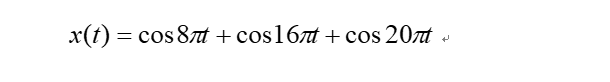这是一个含有三个频率成分的模拟信号，频率分别为选择采样频率Fs=64Hz，对DFT变换点数N分别为：16、32、64这三种情况进行频谱分析，分别打印频谱特性曲线。对三种点数的频谱分析结果进行讨论分析。整个频谱分析过程通过Matlab软件进行程序设计实现。以下为Matlab的程序实现：

function e_6_2
global T;%全局变量 采样周期
Fs=64;T=1/Fs;%Fs采样频率
n=0:64-1;nT=n*T;%采样点数
X3n=cos(8*pi*nT)+cos(16*pi*nT)+cos(20*pi*nT);%采样
subplot(311);fft_stem(X3n,16);%对N=16的频谱分析
subplot(312);fft_stem(X3n,32);%对N=32的频谱分析
subplot(313);fft_stem(X3n,64);%对N=64的频谱分析
end
function fft_stem(A,N) % 计算FFT并打印子函数
global T;Tp=N*T;F=1/Tp;%Tp 观察时间 F 频率分辨率
stem([-N/2:N/2-1]*F,abs(fftshift(fft(A,N))));xlabel('f(Hz)');
%[-N/2:N/2-1]*F产生DFT对应采样点频率（fftshift()零点居中,关于中心反转）
ylabel('幅度');title([num2str(N),'点DFT[X_3(n)]']);xlim([-N*F/2,N*F/2-1]);%x范围
end

三、实验结果分析

（一）序列结果分析

通过对 和 频谱特性曲线结果的比较与分析（为了便于观察频谱和读取频率值对数据进行归一化处理，即分析以为横坐标），可以得出当FFT变换点数N为8 时， 和 频谱特性相同(见图3中的b1与b2)，而N为16时， 和 频谱特性曲线不相同(见图3中的c1与c2)。出现这两种不同情况的原因为 和 为序列为8的有限长序列（如图3的a1和a2）当取N为8时，将两个序列做周期延拓后发现 两个周期序列的波形仅存在相位上的差别，将 向右移动4个单位长度即可得到 ，即，因而两序列的频谱特性相等，而当取N为16时，原 和 序列通过补零的方式变为序列长度为16的序列。此时序列波形完全不同，所以频谱特性必然不同。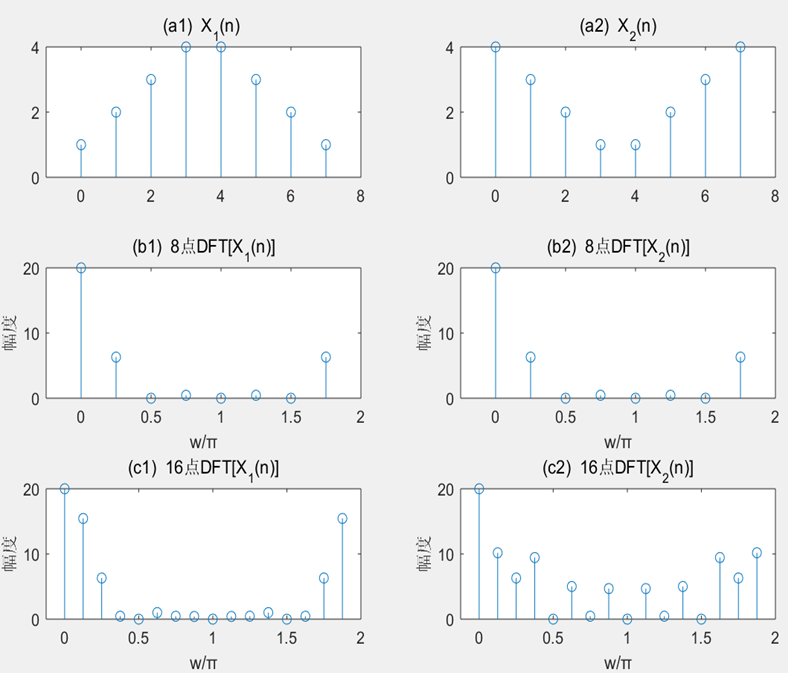（二）模拟信号结果分析

x_3(n) 共有3个频率成分，f1=4Hz, f2 =8Hz, f3=10Hz。所以x_3(n)的周期为0.5s。采样的频率为 Fs=64Hz=16f1=8f2=6.4f3。N为16时，观察时间Tp=16T=0.25s,T为Fs的倒数。不是x_3(n)周期的整数倍，出现混叠现象，严重失真，所以得到的频谱不正确（如图4中a3）。当N=32或64时，观察时间Tp=0.5s或1s, 是周期的整数倍，所以会得到正确的频谱（如图4中b3和c3）,频谱中有3根谱线正好对应位于4Hz，8Hz和10Hz。而N为64时的频谱幅度为40正好为N为32时频谱幅度为20的两倍，即验证DFT对中期序列谱分析的理论。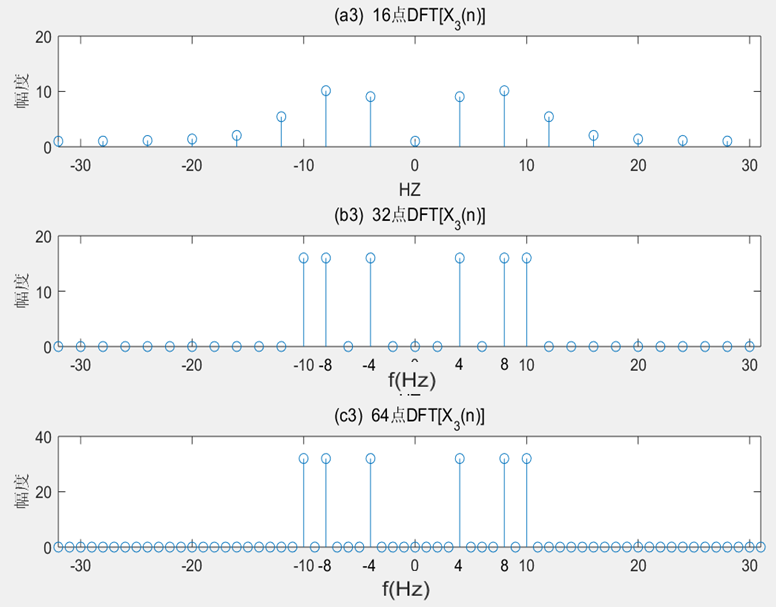点击下载资源

展开全文• 数字信号处理实验，DFT和FFT实验步骤与实例，希望给你在数字信号处理实验中带来帮助
• 本文档为数字信号处理课程设计，fft分析仪，实现了对从声卡采集的声音信号的实时显示，放大缩小，滤波处理，GUI界面的制作，包含详细的程序资料以及matlab仿真结果。
• 这个程序是用C#语言编写的主要实现数字信号处理FFT算法
• 对于fft的应用，在学习了数字信号处理之后，用verilog在底层实现，对fft的应用有了更深刻的了解。
• 对于信号处理来讲，傅里叶变换是从另一个角度观察这个世界的大门。傅里叶变换让我们对于信号的组成与本质有了更深的理解。这些废话就不用再说了，总之，傅里叶变换很重要。不管是对于哪个领域来讲。对于我们学电的人...
• 该篇是FPGA数字信号处理的第9篇，选题为DSP系统中极其常用的FFT运算。上篇介绍了Quartus环境下FFT IP核的使用“FPGA数字信号处理（八）Quartus FFT IP核实现...FPGA Vivado Verilog
• 西安交通大学数字信号处理-快速傅里叶变换FFT实验报告
• 数字信号处理实验&matlab实现FFT算法 FFT matlab 倒码算法 数字信号处理 实验
• FFT是离散傅立叶变换的快速算法，可以将一个信号变换到频域。有些信号在时域上是很难看出什么特征的，但是如果变换到频域之后，就很容易看出特征了。这就是很多信号分析采用FFT变换的原因。另外，FFT可以将一个信号...
• 里面有两份常见数字信号处理算法的C实现代码，适合做DSP或者信号采集处理的工程师参考，或者对数字信号处理感兴趣的同行们
• 引言：FFT(快速傅里叶变换)在雷达信号处理、图像处理、无线通信等行业有着广泛的应用。本文及后续几篇文章，我们针对Xilinx的FFT IP核v9.0提供详细介绍。本文作为开篇，介绍以下内容：Xilinx FFT IP核的特性Xilinx ...
• 数字信号处理dit-fft和ifft的c语言实现...